# Saxon Algebra 2: Other Types of Equations Chapter Exam

Exam Instructions:

Choose your answers to the questions and click 'Next' to see the next set of questions. You can skip questions if you would like and come back to them later with the yellow "Go To First Skipped Question" button. When you have completed the practice exam, a green submit button will appear. Click it to see your results. Good luck!

### Page 2

#### Question 10 10. Which of the following are the solutions to the equation below?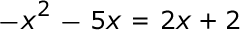### Page 3

#### Question 12 12. Solve the exponential equation below for x: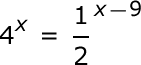#### Question 15 15. Solve the exponential equation below for x: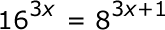### Page 4

#### Question 16 16. Solve the equation.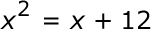#### Question 17 17. The following differential equation represents the change in population as a function of time. What does the 0.03 represent in this equation?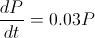### Page 5

#### Question 22 22. Solve the differential equation for your bank balance given as follows.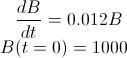### Page 6

#### Question 28 28. Rewrite the quadratic below in standard form: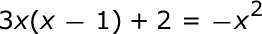#### Question 29 29. Find the roots of the equation.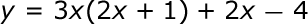#### Question 30 30. Solve the exponential equation below for x: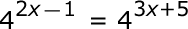#### Saxon Algebra 2: Other Types of Equations Chapter Exam Instructions

Choose your answers to the questions and click 'Next' to see the next set of questions. You can skip questions if you would like and come back to them later with the yellow "Go To First Skipped Question" button. When you have completed the practice exam, a green submit button will appear. Click it to see your results. Good luck!

Support